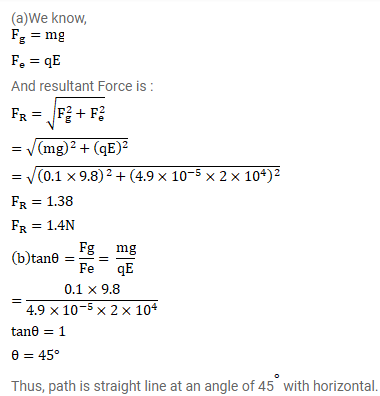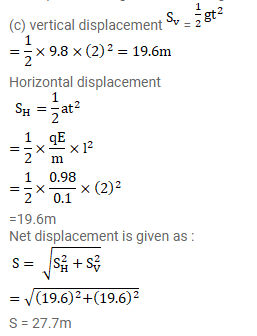# A ball of mass 100g and having a charge ofQuestion:

A ball of mass $100 \mathrm{~g}$ and having a charge of $4.9^{\times 10^{-5}} \mathrm{C}$ is released from rest in a region where a horizontal electric filed of $2.0^{\times 10^{4}} \mathrm{~N} / \mathrm{C}$ exists.

(a) Find the resultant force acting on the ball.

(b) What will be the path of the ball?

(c) Where will the ball be at the end of $2 \mathrm{~s}$ ?

Solution: